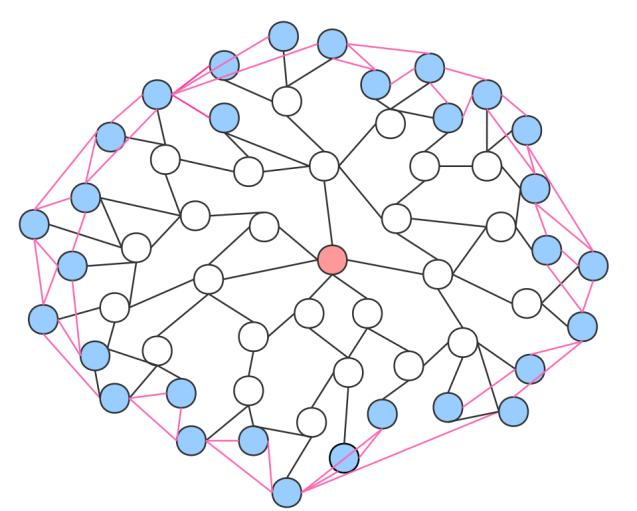# 【图论】Spfa算法求最短路（长得像Dijkstra的，Bellman_Ford的优化算法）图论与数据结构 同时被 2 个专栏收录24 篇文章 1 订阅

### 三、代码实现基础Spfa

给定一个 n 个点 m 条边的有向图，图中可能存在重边和自环， 边权可能为负数。

1≤n,m≤1e5,

3 3
1 2 5
2 3 -3
1 3 4

2


1.链式前向星存图（链式前向星
2.作者重写的memset函数（“#define mem(a,b) memset(a,b,sizeof(a))”）
3.BFS宽度优先搜索的基本思想
4.C++STL中queue队列数据结构的基本用法

//#pragma GCC optimize(2)
#include<iostream>
#include<iomanip>
#include<cstdio>
#include<string>
#include<algorithm>
#include<cmath>
#include<queue>
#include<vector>
#include<map>
#include<stack>
#include<set>
#include<bitset>
#include<ctime>
#include<cstring>
#include<list>
#define ll long long
#define ull unsigned long long
#define INF 0x3f3f3f3f
#define mem(a,b) memset(a,b,sizeof(a))
using namespace std;
typedef  pair<int, int> PII;
const int N = 1e5 + 7;

int e[N], ne[N], w[N], h[N], id = 1;  //链式前向星
int n, m;
int dis[N];   //dis[i]代表从起点到i的距离
bool ch[N];   //用来判断一个点是否在队列中
void add(int a, int b, int c)   //加边函数
{
e[id] = b;
w[id] = c;
ne[id] = h[a];
h[a] = id;
id++;
}

int spfa()
{
mem(dis, 0x3f);   //距离的初始化
dis = 0;   //起点到自己的距离为0
queue<int>q;
q.push(1);   //先把起点放进去
ch = true;   //记录一下起点放进去了
while (!q.empty())   //循环条件：队列非空
{
int f = q.front();   //取出队首元素
q.pop();   //把队首踢出去
ch[f] = false;   //记录一下队首已经被踢出去了
for (int i = h[f]; i != -1; i = ne[i])   //链式前向星遍历所有以f为起点的边
{
int j = e[i];
if (dis[j] > dis[f] + w[i])   //如果点j发生了更新
{
dis[j] = dis[f] + w[i];   //更新一下
if (!ch[j])   //如果点j没在队列中
{
ch[j] = true;   //把点j放进队列
q.push(j);
}
}
}
}
return dis[n];   //输出最后的结果
}

void solve()
{
mem(h, -1);   //初始化链式前向星

cin >> n >> m;
for (int i = 0; i < m; i++)
{
int a, b, c;
cin >> a >> b >> c;
}
int t = spfa();
if (t == INF)   //如果是INF代表到不了
cout << "impossible" << endl;
else
cout << t << endl;
}

int main()
{
//std::ios::sync_with_stdio(false);
//cin.tie(0), cout.tie(0);
solve();
return 0;
}


### 四、代码实现Spfa对负权环的判断

给定一个 n 个点 m 条边的有向图，图中可能存在重边和自环， 边权可能为负数。

1≤n≤2000,
1≤m≤10000,

3 3
1 2 -1
2 3 4
3 1 -4

Yes


//#pragma GCC optimize(2)
#include<iostream>
#include<iomanip>
#include<cstdio>
#include<string>
#include<algorithm>
#include<cmath>
#include<queue>
#include<vector>
#include<map>
#include<stack>
#include<set>
#include<bitset>
#include<ctime>
#include<cstring>
#include<list>
#define ll long long
#define ull unsigned long long
#define INF 0x3f3f3f3f
#define mem(a,b) memset(a,b,sizeof(a))
using namespace std;
typedef  pair<int, int> PII;
const int N = 1e6 + 7;

int e[N], ne[N], w[N], h[N], id = 1;  //链式前向星
int n, m;
int dis[N];   //dis[i]代表从起点到i的距离
bool ch[N];   //用来判断一个点是否在队列中
int num[N];  //num[i]代表走到i经过了多少条边

void add(int a, int b, int c)   //加边函数
{
e[id] = b;
w[id] = c;
ne[id] = h[a];
h[a] = id;
id++;
}

int spfa()
{
/*本题不是求距离，可以不用初始化dis*/
queue<int>q;
for (int i = 1; i <= n; i++)
{
q.push(i);
ch[i] = true;
}
while (!q.empty())   //循环条件：队列非空
{
int f = q.front();   //取出队首元素
q.pop();   //把队首踢出去
ch[f] = false;   //记录一下队首已经被踢出去了
for (int i = h[f]; i != -1; i = ne[i])   //链式前向星遍历所有以f为起点的边
{
int j = e[i];
if (dis[j] > dis[f] + w[i])   //如果点j发生了更新
{
dis[j] = dis[f] + w[i];   //更新一下
num[j] = num[f] + 1;
if (num[j] >= n)  //经过的边的数量如果大于了n-1，那就说明至少重复走了一个点，有负权环（只有存在负权环，才可能在求最短路的时候两次经过一个点）
return -1;
if (!ch[j])   //如果点j没在队列中
{
ch[j] = true;   //把点j放进队列
q.push(j);
}
}
}
}
return 1;   //输出最后的结果
}

void solve()
{
mem(h, -1);   //初始化链式前向星

cin >> n >> m;
for (int i = 0; i < m; i++)
{
int a, b, c;
cin >> a >> b >> c;
}
int t = spfa();
if (t == -1)
cout << "Yes" << endl;
else
cout << "No" << endl;
}

int main()
{
//std::ios::sync_with_stdio(false);
//cin.tie(0), cout.tie(0);
solve();
return 0;
}



11-1828007-30558
09-2628
05-2719
10-0133
08-12236
11-14492
10-2753
07-271万+
09-075万+
09-0515
08-0665
12-0133
11-1812
03-04102
05-14312
02-24148点击重新获取扫码支付余额充值